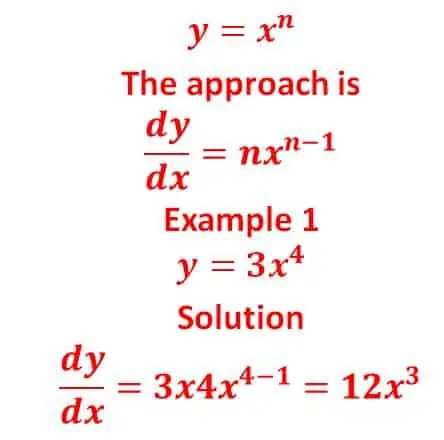0
62

# 4 Hidden Facts In JAMB Mathematics For Candidates

One of the JAMB subjects that candidates are a little bit afraid of is Mathematics. The primary reason is that JAMB mathematics has some basic further-mathematics in the question. And if you are not so sound in it, you are already at a disadvantage.However, there are some hidden facts that you need to understand in JAMB mathematics that will enable you to score high in it. For this reason, I have decided to shed some lights on hidden facts in JAMB mathematics.

## Hidden truths in JAMB Mathematics

1. Learn differentiation, integration, matrix, permutation & combination, and polynomial at foundation level

The topics I just mentioned are simple because they have already made formulas which will enable you to solve any question on them. Don’t let anyone scare you since JAMB will only bring out simple questions from these topics.

1. Make sure you master logarithms & indices, surd, circle geometry, probability, set, number base, mean, median and mode well

These are essential topics you need to work more on to increase your chance of scoring high in JAMB mathematics. They are simple and easy to solve if can practice them as many times as possible

READ HERE  How to Write MM, MMV, MMVIII Roman Numerals In Numbers

JAMB mathematics is different from other subject being that it can waste your time if you don’t know how to manage your time. Most times I do advise candidates who aren’t fantastic in mathematics to make it the last subject to answer. However, you can find some JAMB online CBT on math that you can practice with before the exam day. Using this approach will help increase your speed.

1. Anybody can score high in it

Some candidates believe that only science students can score high in mathematics, but this assertion is false. A social science student can do well in this subject if he/she prepares well and follow the first 3 points that I give above.

## JAMB Mathematics Question and Answer

Question 1

Tanθ = 5/4, find sin2θ – cos2θ

A. 41/9     B. 9/41     C. 1       D. 5/4

Recall,

Opposite = 5

Also,

(Hypotenuse)2 = 52 + 42 = 25 + 16 = 41

sin2θ = (opposite)2/(hypotenuse)2 = 25/41

sin2θ – cos2θ = 25/41 – 16/41 = 9/41

Question 2

In triangle XYZ, <XYZ = 15o, <XZY = 45o, |XY| = 7cm, find |YZ|

A. 7√2 cm    B. 7cm    C. 14√2 cm       D. 7/2 √6 cm

READ HERE  Make a the Subject Of The Formula v = u + at [Solved]

To calculate, use sine rule

|XY|/sine (<XZY) = |YZ| / sine (<YXZ)

Note,

<YXZ + <XZY +  <XYZ = 180 (sum of angles in a triangle)

<YXZ = 180 – 15 – 45 = 120

7/sine 45 = |YZ| / sine 120

sine 45 = 1/√2

sine 120 = √3 /2

7√2 = 2 |YZ| / √3

7√6 / 2 = |YZ|

|YZ| = 7/2 √6 cm

Question 3

The mean of the numbers 3, 6, 4, x, and 7 is 5. Find the standard deviation

A. 2         B. 3    C. √3   D. √2

Mean = (3 + 6 + 4 + x + 7) /5

5 = (3 + 6 + 4 + x + 7) /5

25 = 20 + x

X = 5

To calculate standard deviation,

((3-5)2 + (6-5)2 + (4-5)2 + (5-5)2 + (7-5)2)/5 =  (4 +1+1+0+4) /5 = 10/5 = 2

Then, find the square root

S.D = √2

Question 4

A cinema hall contains a certain number of people. If 22 ½ % are children, 47 ½% are men and 84 are women. Find the number of men in the hall.

A. 133      B. 113     C. 63      D. 84

Percentage of children + men + women = 100

Let x be the percentage of women

22 ½ % + 47 ½%  + x = 100

X = 100 – 70 = 30%

Let the total number of people in the cinema hall be Y

30% of Y = 84 for women

i.e.

30/100 x Y = 84

8400/30 = Y

Y = 280

Number of men,

47 ½% of 280

95/200 x 280 = 133

READ HERE  XXXVII, CXXXIX, XXIX, XXVI, LXXX, XL, XI Roman Numerals In Numbers

Question 5

Find the derivative of y = sin2(5x) with respect to x

A. 2sin5xcos5x       B. 5sin5xcos5x   C. 10sin5xcos5x   D. 15sin5xcos5x

Y = (sin(5x))2

Let u = sin5x

Let a = 5x

Da/dx = 5

U = sina

Du/da = cos a

Du/dx = da/dx x du/da = 5 x cosa = 5cosa = 5cos5x

Y = u2

Dy/du = 2u

So,

Dy/dx = dy/du x du/dx = 5cos5x x 2u = 10ucos5x

Since u = sin5x

Dy/dx = 10sin5xcos5x